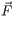🤓 Based on our data, we think this question is relevant for Professor Maheswari's class at BC.

###### FREE Expert Solution

Part A

Average Power:

$\overline{){{\mathbf{P}}}_{{\mathbf{avg}}}{\mathbf{=}}\frac{{\mathbf{W}}_{\mathbf{F}}}{\mathbf{t}}}$###### Problem Details

A sled of mass m is being pulled horizontally by a constant horizontal force of magnitude F. The coefficient of kinetic friction is μk . During time interval t, the sled moves a distance s, starting from rest.

Part A

Find the average power Pavg created by the force F. Express your answer in terms of the given quantities and, if necessary, appropriate constants. You may or may not use all of the given quantities.

Part B

Find the average velocity Vavg of the sled during that time interval. Express your answer in terms of the given quantities and, if necessary, appropriate constants. You may or may not use all of the given quantities.

Part C

Find the average power Pavg created by the force F in terms of the average speed Vavg of the sled. Express your answer in terms of F and Vavg.

You just obtained a very useful formula for the average power:

If an object is moving at a constant speed, and the force F is also constant, this formula can be used to find the average power. If v is changing, the formula can be used to find the instantaneous power at any given moment (with the quantity v in this case meaning the instantaneous velocity, of course).

Part D

A sled of mass m is being pulled horizontally by a constant upward force of magnitude F that makes an angle θ with the direction of motion. The coefficient of kinetic friction is μk. The average velocity of the sled is Vavg.

Find the average power Pavg created by force F.

Let us now consider several questions that include numeric data.

A sled is being pulled along a horizontal surface by a horizontal forceof magnitude 600 N. Starting from rest, the sled speeds up with acceleration 0.08 m/s2 for 1 minute.

Part E

Find the average power Pavg created by force.

Part F

Find the instantaneous power P created by forceat t = 10 s.

P = _____ W

Part G

Find the instantaneous power P created by the normal force at t = 10 s. The magnitude of the acceleration due to gravity is g = 9.8 m/s2.